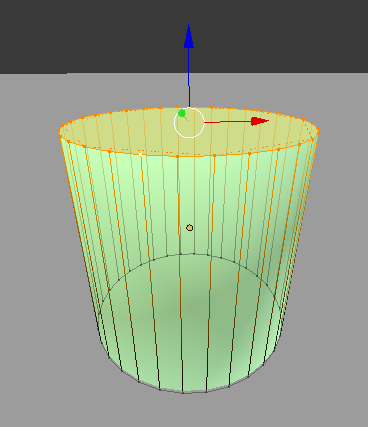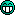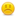Hello,

im looking for a script that would find the radius and maybe the height of this selection?thanksno help?for blender this isn’t a geometric volume, so there’s no easy way to provide a function to calculate a radius from arbitrary selections. You could however select two opposing verts, measure the distance and divide by 2.

thank you but how would i measure the distance?

This assumes your vertices form a circle approximately. Otherwise getting a radius doesn’t make much sense anyway. They need to be fairly planar as well but do not have to sit on the xy-plane. Any angle will work. First it finds the middle point, then measures the distance of each vertex from that point and finally averages those distances. Result is printed to the console (window->toggle system console).

# This example assumes we have a mesh object in edit-mode

import bpy
import bmesh
from mathutils import Vector

# Get the active mesh and get bmesh data

obj = bpy.context.edit_object
me = obj.data
bm = bmesh.from_edit_mesh(me)

#get selection
selected=[v for v in bm.verts if v.select]
#get middlepoint
vsum=Vector() #zero vector
for v in selected: vsum+=v.co #sum all selected vectors together
midPoint=vsum/len(selected) #average point

#get average distance from middlepoint, to account for minor variability.
distances=[(v.co-midPoint).length for v in selected]
averageDist=sum(distances)/len(distances)

print(averageDist)

here is a version with more data printed on console

``````

rint ()
print ()

# This example assumes we have a mesh object in edit-mode

import bpy
import bmesh

from mathutils import Vector

# Get the active mesh and get bmesh data

obj = bpy.context.edit_object
me = obj.data
bm = bmesh.from_edit_mesh(me)

# get selection
selected=[v for v in bm.verts if v.select]
# get middlepoint
vsum=Vector()         # zero vector

for v in selected: vsum+=v.co     # sum all selected vectors together
midPoint=vsum/len(selected)      # average point

print('mid point= ',midPoint)
print ()

# get average distance from middlepoint, to account for minor variability.

distances=[(v.co-midPoint).length for v in selected]

print ()
print('distance  = ',distances )
print ()
print ('Qty points =',len(distances))
print ()
averageDist=sum(distances)/len(distances)

print ()
print (' end ')
print ()

``````

is it possible to automaticaly select all the verts instead of manualy doing this

also how do you contrl-A the object before
cause this could change the values i guess!

thanks

I don’t quite understand the first question. There’s no magic way for you script to know which vertices you wish to use, unless you are only interested in some simple special cases like cylinders in default alignment.

And yes, this calculation is done in local space, so scaling does affect the result. Rotatation and translation should not affect it though. Apply scaling before using it if you need the result in world space. Off the top of my head I don’t know how to do that in a script. If you can’t do that at all, or don’t want to, for whatever reason, you can multiply all the v.co values by the object’s world matrix.

may depends on selected verts
but would be interesting for a whole object like circle !

the ctlr-a i thinkj can be done with this
bpy.ops.object.transform_apply(location=True, rotation=True, scale=True)

but don’t know the bpy equivalent !

thanks

Here’s an implementation of my proposal:

``````import bpy, bmesh

class VertDistance(bpy.types.Operator):
"""Tooltip"""
bl_idname = "mesh.vert_distance"
bl_label = "Half distance of 2 verts"

@classmethod
def poll(cls, context):
return (context.active_object is not None and
context.active_object.type == 'MESH' and
context.active_object.mode == 'EDIT')

def execute(self, context):
ob = context.active_object
ob_mat = ob.matrix_world
me = ob.data

bm = bmesh.from_edit_mesh(me)
verts = [v for v in bm.verts if v.select]
if len(verts) != 2:
self.report({'ERROR'}, "Need 2 selected vertices!")
return {'CANCELLED'}

v1, v2 = verts
radius = (ob_mat * v2.co - ob_mat * v1.co).length / 2
return {'FINISHED'}

def register():
bpy.utils.register_class(VertDistance)

def unregister():
bpy.utils.unregister_class(VertDistance)

if __name__ == "__main__":
register()

``````

Oh, do you mean you simply want to use all vertices in the object? In that case you can just use
selection=me.vertices (I think)
and remove all the bmesh stuff. You don’t need to be in edit mode either. Your object needs to be fairly uniformly circular though. If you have more vertices on one side than the other, you can get skewed results.

here is one for all verts in one object

``````

rint ()
print ()

# This example assumes we have a mesh object in edit-mode

import bpy
import bmesh

from mathutils import Vector

# Get the active mesh and get bmesh data

bpy.ops.object.transform_apply(location=True, rotation=True, scale=True)

obj = bpy.context.active_object

me = obj.data

print(' me.vertices =', me.vertices,' len =',len(me.vertices))

selection=me.vertices

# get middlepoint
vsum=Vector()         # zero vector

for v in selection: vsum+=v.co

midPoint=vsum/len(selection)      # average point

print('mid point= ',midPoint)
print ()

# get average distance from middlepoint, to account for minor variability.

distances=[(v.co-midPoint).length for v in selection]

print ()
print('distance  = ',distances )
print ()
print ('Qty points =',len(distances))
print ()
averageDist=sum(distances)/len(distances)

print ()
print (' end ')
print ()

``````

i would not use a destructive method in such a script

so drop
bpy.ops.object.transform_apply(location=True, rotation=True, scale=True)

and and multiply Object.matrix_world with Vertex.co

why do you say it is a destructive method?

many times you have to Cltr-A objects to get right dimensions!

but also itneresting to do it with world matrix

how do you write this new line for world matrix

thanks

sorry, i was going to say “don’t use a destructive method”

transform_apply is sort of destructive, 'cause it applies the transformations and therefore changes the mesh data itself.

non-destructive way:

``````import bpyfrom mathutils import Vector

ob = bpy.context.active_object
me = ob.data

selection = me.vertices

vsum = Vector() # zero vector

for v in selection:
vsum += v.co # we can use local coord here

midPoint = vsum / len(selection) # average point
midPoint = ob.matrix_world * midPoint # now turn into global coord

distances=[(ob.matrix_world * v.co - midPoint).length for v in selection]
averageDist = sum(distances) / len(distances)

print(averageDist)
``````

thanks everyone